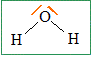1) lewis representation of butanol                                                                        lewis representation of water :2)  M(h2O) = 18g/mol n(h2O) = m(h2O) / M(h2O) n(h2O)=1000/18= 55.55 mol M(C4H10O)= 74 n=m/M =2000/74 =27 MOL In 1kg of butanol, there are 55.5 mol In 2kg of water, there are 27 mol. 3) equation :C3H8+  ...O2--> ....CO2+....H2O the equation complete is : C3H8+  5O2-->3CO2+4H2O